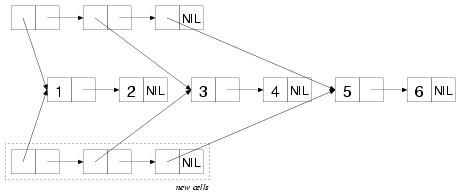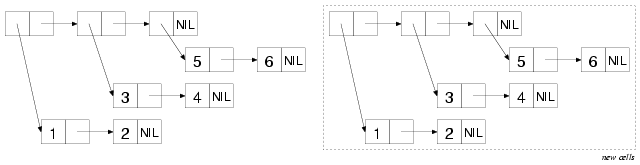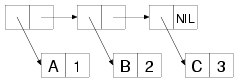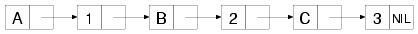# 13. Beyond Lists: Other Uses for Cons Cells

As you saw in the previous chapter, the list data type is an illusion created by a set of functions that manipulate cons cells. Common Lisp also provides functions that let you treat data structures built out of cons cells as trees, sets, and lookup tables. In this chapter I'll give you a quick tour of some of these other data structures and the functions for manipulating them. As with the list-manipulation functions, many of these functions will be useful when you start writing more complicated macros and need to manipulate Lisp code as data.

## Trees

Treating structures built from cons cells as trees is just about as natural as treating them as lists. What is a list of lists, after all, but another way of thinking of a tree? The difference between a function that treats a bunch of cons cells as a list and a function that treats the same bunch of cons cells as a tree has to do with which cons cells the functions traverse to find the values of the list or tree. The cons cells traversed by a list function, called the list structure, are found by starting at the first cons cell and following `CDR` references until reaching a `NIL`. The elements of the list are the objects referenced by the `CAR`s of the cons cells in the list structure. If a cons cell in the list structure has a `CAR` that references another cons cell, the referenced cons cell is considered to be the head of a list that's an element of the outer list.1 Tree structure, on the other hand, is traversed by following both `CAR` and `CDR` references for as long as they point to other cons cells. The values in a tree are thus the atomic--non-cons-cell-values referenced by either the `CAR`s or the `CDR`s of the cons cells in the tree structure.

For instance, the following box-and-arrow diagram shows the cons cells that make up the list of lists: `((1 2) (3 4) (5 6))`. The list structure includes only the three cons cells inside the dashed box while the tree structure includes all the cons cells.To see the difference between a list function and a tree function, you can consider how the functions `COPY-LIST` and `COPY-TREE` will copy this bunch of cons cells. `COPY-LIST`, as a list function, copies the cons cells that make up the list structure. That is, it makes a new cons cell corresponding to each of the cons cells inside the dashed box. The `CAR`s of each of these new cons cells reference the same object as the `CAR`s of the original cons cells in the list structure. Thus, `COPY-LIST` doesn't copy the sublists `(1 2)`, `(3 4)`, or `(5 6)`, as shown in this diagram:`COPY-TREE`, on the other hand, makes a new cons cell for each of the cons cells in the diagram and links them together in the same structure, as shown in this diagram:Where a cons cell in the original referenced an atomic value, the corresponding cons cell in the copy will reference the same value. Thus, the only objects referenced in common by the original tree and the copy produced by `COPY-TREE` are the numbers 1-6, and the symbol `NIL`.

Another function that walks both the `CAR`s and the `CDR`s of a tree of cons cells is `TREE-EQUAL`, which compares two trees, considering them equal if the tree structure is the same shape and if the leaves are `EQL` (or if they satisfy the test supplied with the `:test` keyword argument).

Some other tree-centric functions are the tree analogs to the `SUBSTITUTE` and `NSUBSTITUTE` sequence functions and their `-IF` and `-IF-NOT` variants. The function `SUBST`, like `SUBSTITUTE`, takes a new item, an old item, and a tree (as opposed to a sequence), along with `:key` and `:test` keyword arguments, and it returns a new tree with the same shape as the original tree but with all instances of the old item replaced with the new item. For example:

```CL-USER> (subst 10 1 '(1 2 (3 2 1) ((1 1) (2 2))))
(10 2 (3 2 10) ((10 10) (2 2)))```

`SUBST-IF` is analogous to `SUBSTITUTE-IF`. Instead of an old item, it takes a one-argument function--the function is called with each atomic value in the tree, and whenever it returns true, the position in the new tree is filled with the new value. `SUBST-IF-NOT` is the same except the values where the test returns `NIL` are replaced. `NSUBST`, `NSUBST-IF`, and `NSUBST-IF-NOT` are the recycling versions of the `SUBST` functions. As with most other recycling functions, you should use these functions only as drop-in replacements for their nondestructive counterparts in situations where you know there's no danger of modifying a shared structure. In particular, you must continue to save the return value of these functions since you have no guarantee that the result will be `EQ` to the original tree.2

## Sets

Sets can also be implemented in terms of cons cells. In fact, you can treat any list as a set--Common Lisp provides several functions for performing set-theoretic operations on lists. However, you should bear in mind that because of the way lists are structured, these operations get less and less efficient the bigger the sets get.

That said, using the built-in set functions makes it easy to write set-manipulation code. And for small sets they may well be more efficient than the alternatives. If profiling shows you that these functions are a performance bottleneck in your code, you can always replace the lists with sets built on top of hash tables or bit vectors.

To build up a set, you can use the function `ADJOIN`. `ADJOIN` takes an item and a list representing a set and returns a list representing the set containing the item and all the items in the original set. To determine whether the item is present, it must scan the list; if the item isn't found, `ADJOIN` creates a new cons cell holding the item and pointing to the original list and returns it. Otherwise, it returns the original list.

`ADJOIN` also takes `:key` and `:test` keyword arguments, which are used when determining whether the item is present in the original list. Like `CONS`, `ADJOIN` has no effect on the original list--if you want to modify a particular list, you need to assign the value returned by `ADJOIN` to the place where the list came from. The modify macro `PUSHNEW` does this for you automatically.

```CL-USER> (defparameter *set* ())
*SET*
(1)
CL-USER> *set*
NIL
CL-USER> (setf *set* (adjoin 1 *set*))
(1)
CL-USER> (pushnew 2 *set*)
(2 1)
CL-USER> *set*
(2 1)
CL-USER> (pushnew 2 *set*)
(2 1)```

You can test whether a given item is in a set with `MEMBER` and the related functions `MEMBER-IF` and `MEMBER-IF-NOT`. These functions are similar to the sequence functions `FIND`, `FIND-IF`, and `FIND-IF-NOT` except they can be used only with lists. And instead of returning the item when it's present, they return the cons cell containing the item--in other words, the sublist starting with the desired item. When the desired item isn't present in the list, all three functions return `NIL`.

The remaining set-theoretic functions provide bulk operations: `INTERSECTION`, `UNION`, `SET-DIFFERENCE`, and `SET-EXCLUSIVE-OR`. Each of these functions takes two lists and `:key` and `:test` keyword arguments and returns a new list representing the set resulting from performing the appropriate set-theoretic operation on the two lists: `INTERSECTION` returns a list containing all the elements found in both arguments. `UNION` returns a list containing one instance of each unique element from the two arguments.3 `SET-DIFFERENCE` returns a list containing all the elements from the first argument that don't appear in the second argument. And `SET-EXCLUSIVE-OR` returns a list containing those elements appearing in only one or the other of the two argument lists but not in both. Each of these functions also has a recycling counterpart whose name is the same except with an N prefix.

Finally, the function `SUBSETP` takes two lists and the usual `:key` and `:test` keyword arguments and returns true if the first list is a subset of the second--if every element in the first list is also present in the second list. The order of the elements in the lists doesn't matter.

```CL-USER> (subsetp '(3 2 1) '(1 2 3 4))
T
CL-USER> (subsetp '(1 2 3 4) '(3 2 1))
NIL```

## Lookup Tables: Alists and Plists

In addition to trees and sets, you can build tables that map keys to values out of cons cells. Two flavors of cons-based lookup tables are commonly used, both of which I've mentioned in passing in previous chapters. They're association lists, also called alists, and property lists, also known as plists. While you wouldn't use either alists or plists for large tables--for that you'd use a hash table--it's worth knowing how to work with them both because for small tables they can be more efficient than hash tables and because they have some useful properties of their own.

An alist is a data structure that maps keys to values and also supports reverse lookups, finding the key when given a value. Alists also support adding key/value mappings that shadow existing mappings in such a way that the shadowing mapping can later be removed and the original mappings exposed again.

Under the covers, an alist is essentially a list whose elements are themselves cons cells. Each element can be thought of as a key/value pair with the key in the cons cell's `CAR` and the value in the `CDR`. For instance, the following is a box-and-arrow diagram of an alist mapping the symbol `A` to the number 1, `B` to 2, and `C` to 3:Unless the value in the `CDR` is a list, cons cells representing the key/value pairs will be dotted pairs in s-expression notation. The alist diagramed in the previous figure, for instance, is printed like this:

`((A . 1) (B . 2) (C . 3))`

The main lookup function for alists is `ASSOC`, which takes a key and an alist and returns the first cons cell whose `CAR` matches the key or `NIL` if no match is found.

```CL-USER> (assoc 'a '((a . 1) (b . 2) (c . 3)))
(A . 1)
CL-USER> (assoc 'c '((a . 1) (b . 2) (c . 3)))
(C . 3)
CL-USER> (assoc 'd '((a . 1) (b . 2) (c . 3)))
NIL```

To get the value corresponding to a given key, you simply pass the result of `ASSOC` to `CDR`.

```CL-USER> (cdr (assoc 'a '((a . 1) (b . 2) (c . 3))))
1```

By default the key given is compared to the keys in the alist using `EQL`, but you can change that with the standard combination of `:key` and `:test` keyword arguments. For instance, if you wanted to use string keys, you might write this:

```CL-USER> (assoc "a" '(("a" . 1) ("b" . 2) ("c" . 3)) :test #'string=)
("a" . 1)```

Without specifying `:test` to be `STRING=`, that `ASSOC` would probably return `NIL` because two strings with the same contents aren't necessarily `EQL`.

```CL-USER> (assoc "a" '(("a" . 1) ("b" . 2) ("c" . 3)))
NIL```

Because `ASSOC` searches the list by scanning from the front of the list, one key/value pair in an alist can shadow other pairs with the same key later in the list.

```CL-USER> (assoc 'a '((a . 10) (a . 1) (b . 2) (c . 3)))
(A . 10)```

You can add a pair to the front of an alist with `CONS` like this:

`(cons (cons 'new-key 'new-value) alist)`

However, as a convenience, Common Lisp provides the function `ACONS`, which lets you write this:

`(acons 'new-key 'new-value alist)`

Like `CONS`, `ACONS` is a function and thus can't modify the place holding the alist it's passed. If you want to modify an alist, you need to write either this:

`(setf alist (acons 'new-key 'new-value alist))`

or this:

`(push (cons 'new-key 'new-value) alist)`

Obviously, the time it takes to search an alist with `ASSOC` is a function of how deep in the list the matching pair is found. In the worst case, determining that no pair matches requires `ASSOC` to scan every element of the alist. However, since the basic mechanism for alists is so lightweight, for small tables an alist can outperform a hash table. Also, alists give you more flexibility in how you do the lookup. I already mentioned that `ASSOC` takes `:key` and `:test` keyword arguments. When those don't suit your needs, you may be able to use the `ASSOC-IF` and `ASSOC-IF-NOT` functions, which return the first key/value pair whose `CAR` satisfies (or not, in the case of `ASSOC-IF-NOT`) the test function passed in the place of a specific item. And three functions--`RASSOC`, `RASSOC-IF`, and `RASSOC-IF-NOT`--work just like the corresponding `ASSOC` functions except they use the value in the `CDR` of each element as the key, performing a reverse lookup.

The function `COPY-ALIST` is similar to `COPY-TREE` except, instead of copying the whole tree structure, it copies only the cons cells that make up the list structure, plus the cons cells directly referenced from the `CAR`s of those cells. In other words, the original alist and the copy will both contain the same objects as the keys and values, even if those keys or values happen to be made up of cons cells.

Finally, you can build an alist from two separate lists of keys and values with the function `PAIRLIS`. The resulting alist may contain the pairs either in the same order as the original lists or in reverse order. For example, you may get this result:

```CL-USER> (pairlis '(a b c) '(1 2 3))
((C . 3) (B . 2) (A . 1))```

Or you could just as well get this:

```CL-USER> (pairlis '(a b c) '(1 2 3))
((A . 1) (B . 2) (C . 3))```

The other kind of lookup table is the property list, or plist, which you used to represent the rows in the database in Chapter 3. Structurally a plist is just a regular list with the keys and values as alternating values. For instance, a plist mapping `A`, `B`, and `C`, to 1, 2, and 3 is simply the list ```(A 1 B 2 C 3)```. In boxes-and-arrows form, it looks like this:However, plists are less flexible than alists. In fact, plists support only one fundamental lookup operation, the function `GETF`, which takes a plist and a key and returns the associated value or `NIL` if the key isn't found. `GETF` also takes an optional third argument, which will be returned in place of `NIL` if the key isn't found.

Unlike `ASSOC`, which uses `EQL` as its default test and allows a different test function to be supplied with a `:test` argument, `GETF` always uses `EQ` to test whether the provided key matches the keys in the plist. Consequently, you should never use numbers or characters as keys in a plist; as you saw in Chapter 4, the behavior of `EQ` for those types is essentially undefined. Practically speaking, the keys in a plist are almost always symbols, which makes sense since plists were first invented to implement symbolic "properties," arbitrary mappings between names and values.

You can use `SETF` with `GETF` to set the value associated with a given key. `SETF` also treats `GETF` a bit specially in that the first argument to `GETF` is treated as the place to modify. Thus, you can use `SETF` of `GETF` to add a new key/value pair to an existing plist.

```CL-USER> (defparameter *plist* ())
*PLIST*
CL-USER> *plist*
NIL
CL-USER> (setf (getf *plist* :a) 1)
1
CL-USER> *plist*
(:A 1)
CL-USER> (setf (getf *plist* :a) 2)
2
CL-USER> *plist*
(:A 2)```

To remove a key/value pair from a plist, you use the macro `REMF`, which sets the place given as its first argument to a plist containing all the key/value pairs except the one specified. It returns true if the given key was actually found.

```CL-USER> (remf *plist* :a)
T
CL-USER> *plist*
NIL```

Like `GETF`, `REMF` always uses `EQ` to compare the given key to the keys in the plist.

Since plists are often used in situations where you want to extract several properties from the same plist, Common Lisp provides a function, `GET-PROPERTIES`, that makes it more efficient to extract multiple values from a single plist. It takes a plist and a list of keys to search for and returns, as multiple values, the first key found, the corresponding value, and the head of the list starting with the found key. This allows you to process a property list, extracting the desired properties, without continually rescanning from the front of the list. For instance, the following function efficiently processes--using the hypothetical function `process-property`--all the key/value pairs in a plist for a given list of keys:

```(defun process-properties (plist keys)
(loop while plist do
(multiple-value-bind (key value tail) (get-properties plist keys)
(when key (process-property key value))
(setf plist (cddr tail)))))```

The last special thing about plists is the relationship they have with symbols: every symbol object has an associated plist that can be used to store information about the symbol. The plist can be obtained via the function `SYMBOL-PLIST`. However, you rarely care about the whole plist; more often you'll use the functions `GET`, which takes a symbol and a key and is shorthand for a `GETF` of the same key in the symbols `SYMBOL-PLIST`.

`(get 'symbol 'key) === (getf (symbol-plist 'symbol) 'key)`

Like `GETF`, `GET` is `SETF`able, so you can attach arbitrary information to a symbol like this:

`(setf (get 'some-symbol 'my-key) "information")`

To remove a property from a symbol's plist, you can use either `REMF` of `SYMBOL-PLIST` or the convenience function `REMPROP`.4

`(remprop 'symbol 'key) === (remf (symbol-plist 'symbol key))`

Being able to attach arbitrary information to names is quite handy when doing any kind of symbolic programming. For instance, one of the macros you'll write in Chapter 24 will attach information to names that other instances of the same macros will extract and use when generating their expansions.

## DESTRUCTURING-BIND

One last tool for slicing and dicing lists that I need to cover since you'll need it in later chapters is the `DESTRUCTURING-BIND` macro. This macro provides a way to destructure arbitrary lists, similar to the way macro parameter lists can take apart their argument list. The basic skeleton of a `DESTRUCTURING-BIND` is as follows:

```(destructuring-bind (parameter*) list
body-form*)```

The parameter list can include any of the types of parameters supported in macro parameter lists such as `&optional`, `&rest`, and `&key` parameters.5 And, as in macro parameter lists, any parameter can be replaced with a nested destructuring parameter list, which takes apart the list that would otherwise have been bound to the replaced parameter. The list form is evaluated once and should return a list, which is then destructured and the appropriate values are bound to the variables in the parameter list. Then the body-forms are evaluated in order with those bindings in effect. Some simple examples follow:

```(destructuring-bind (x y z) (list 1 2 3)
(list :x x :y y :z z)) ==> (:X 1 :Y 2 :Z 3)

(destructuring-bind (x y z) (list 1 (list 2 20) 3)
(list :x x :y y :z z)) ==> (:X 1 :Y (2 20) :Z 3)

(destructuring-bind (x (y1 y2) z) (list 1 (list 2 20) 3)
(list :x x :y1 y1 :y2 y2 :z z)) ==> (:X 1 :Y1 2 :Y2 20 :Z 3)

(destructuring-bind (x (y1 &optional y2) z) (list 1 (list 2 20) 3)
(list :x x :y1 y1 :y2 y2 :z z)) ==> (:X 1 :Y1 2 :Y2 20 :Z 3)

(destructuring-bind (x (y1 &optional y2) z) (list 1 (list 2) 3)
(list :x x :y1 y1 :y2 y2 :z z)) ==> (:X 1 :Y1 2 :Y2 NIL :Z 3)

(destructuring-bind (&key x y z) (list :x 1 :y 2 :z 3)
(list :x x :y y :z z)) ==> (:X 1 :Y 2 :Z 3)

(destructuring-bind (&key x y z) (list :z 1 :y 2 :x 3)
(list :x x :y y :z z)) ==> (:X 3 :Y 2 :Z 1)```

One kind of parameter you can use with `DESTRUCTURING-BIND` and also in macro parameter lists, though I didn't mention it in Chapter 8, is a `&whole` parameter. If specified, it must be the first parameter in a parameter list, and it's bound to the whole list form.6 After a `&whole` parameter, other parameters can appear as usual and will extract specific parts of the list just as they would if the `&whole` parameter weren't there. An example of using `&whole` with `DESTRUCTURING-BIND` looks like this:

```(destructuring-bind (&whole whole &key x y z) (list :z 1 :y 2 :x 3)
(list :x x :y y :z z :whole whole))
==> (:X 3 :Y 2 :Z 1 :WHOLE (:Z 1 :Y 2 :X 3))```

You'll use a `&whole` parameter in one of the macros that's part of the HTML generation library you'll develop in Chapter 31. However, I have a few more topics to cover before you can get to that. After two chapters on the rather Lispy topic of cons cells, you can now turn to the more prosaic matter of how to deal with files and filenames.

1It's possible to build a chain of cons cells where the `CDR` of the last cons cell isn't `NIL` but some other atom. This is called a dotted list because the last cons is a dotted pair.

2It may seem that the `NSUBST` family of functions can and in fact does modify the tree in place. However, there's one edge case: when the "tree" passed is, in fact, an atom, it can't be modified in place, so the result of `NSUBST` will be a different object than the argument: `(nsubst 'x 'y 'y) X`.

3`UNION` takes only one element from each list, but if either list contains duplicate elements, the result may also contain duplicates.

4It's also possible to directly ```SETF SYMBOL-PLIST```. However, that's a bad idea, as different code may have added different properties to the symbol's plist for different reasons. If one piece of code clobbers the symbol's whole plist, it may break other code that added its own properties to the plist.

5Macro parameter lists do support one parameter type, `&environment` parameters, which `DESTRUCTURING-BIND` doesn't. However, I didn't discuss that parameter type in Chapter 8, and you don't need to worry about it now either.

6When a `&whole` parameter is used in a macro parameter list, the form it's bound to is the whole macro form, including the name of the macro.